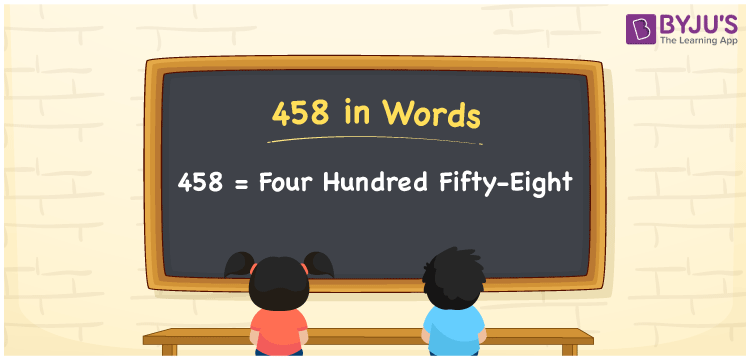# 458 in Words

458 in words can be written as Four Hundred Fifty-Eight. The place value chart discussed here helps in writing the numbers in words. If you offer food for Rs. 458 to a kid, then you can say that “I offered Four Hundred Fifty-Eight Rupees to a kid”. The experts at BYJU’S provide accurate information regarding the numbers in words concept to help students ace the exams. Hence, 458 can be read as “Four Hundred Fifty-Eight” in words.

 458 in words Four Hundred Fifty-Eight Four Hundred Fifty-Eight in Numbers 458

## 458 in English Words## How to Write 458 in Words?

As discussed previously, you will be able to convert the numbers into words using the English alphabet from the place value chart below.

 Hundreds Tens Ones 4 5 8

The expanded form of 458 is provided below:

4 x Hundred + 5 × Ten + 8 × One

= 4 x 100 + 5 x 10 + 8 x 1

= 400 + 50 + 8

= 458

= Four Hundred Fifty-Eight

Therefore, 458 in words is written as Four Hundred Fifty-Eight.

458 is a natural number that precedes 459 and succeeds 457.

458 in words – Four Hundred Fifty-Eight

Is 458 an odd number? – No

Is 458 an even number? – Yes

Is 458 a perfect square number? – No

Is 458 a perfect cube number? – No

Is 458 a prime number? – No

Is 458 a composite number? – Yes

## Frequently Asked Questions on 458 in Words

Q1

### How do you write the number 458 in words?

458 in words is Four Hundred Fifty-Eight.
Q2

### Find the value of 500 minus 42.

The value of 500 minus 42 is 458. Therefore, 458 in words is Four Hundred Fifty-Eight.
Q3

### Is 458 a perfect square?

No, 458 is not a perfect square as it cannot be expressed as the product of two integers which are equal.